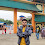# HackerRank Structuring the Document solution in c programming

In this HackerRank Structuring the document in c programming problem solution A document is represented as a collection paragraphs, a paragraph is represented as a collection of sentences, a sentence is represented as a collection of words and a word is represented as a collection of lower-case ([a-z]) and upper-case ([A-Z]) English characters. You will convert a raw text document into its component paragraphs, sentences and words. To test your results, queries will ask you to return a specific paragraph, sentence or word as described below.

Alicia is studying the C programming language at the University of Dunkirk and she represents the words, sentences, paragraphs, and documents using pointers:

A word is described by:

struct word {

char* data;

};

A sentence is described by:

struct sentence {

struct word* data;

int word_count;//the number of words in a sentence

};

The words in the sentence are separated by one space (" "). The last word does not end with a space.

A paragraph is described by:

struct paragraph {

struct sentence* data  ;

int sentence_count;//the number of sentences in a paragraph

};

The sentences in the paragraph are separated by one period (".").

A document is described by:

struct document {

struct paragraph* data;

int paragraph_count;//the number of paragraphs in a document

};

The paragraphs in the document are separated by one newline("\n"). The last paragraph does not end with a newline.

## HackerRank Structuring the document problem solution in c programming.

```#include <stdio.h>
#include <stdlib.h>
#include <string.h>
#include <assert.h>
#define MAX_CHARACTERS 1005
#define MAX_PARAGRAPHS 5

struct word {
char* data;
};

struct sentence {
struct word* data;
int word_count;//denotes number of words in a sentence
};

struct paragraph {
struct sentence* data  ;
int sentence_count;//denotes number of sentences in a paragraph
};

struct document {
struct paragraph* data;
int paragraph_count;//denotes number of paragraphs in a document
};

struct word get_word(char* text, int beg, int end) {
answer.data = calloc(end - beg + 2, sizeof(char));
int index = 0;
int i;
for (i = beg; i <= end; i++)
}

struct sentence get_sentence(char* text, int beg, int end) {
int i;
for (i = beg; i <= end; i++)
if (text[i] == ' ')
int start = beg;
int index = 0;
for (i = beg; i <= end; i++)
if (text[i] == ' ')
{
answer.data[index++] = get_word(text, start, i - 1);
start = i + 1;
}
answer.data[index] = get_word(text, start, i - 1);
}

struct paragraph get_paragraph(char* text, int beg, int end) {
int i;
for (i = beg; i <= end; i++)
if (text[i] == '.')
int start = beg;
int index = 0;
for (i = beg; i <= end; i++)
if (text[i] == '.')
{
answer.data[index++] = get_sentence(text, start, i - 1);
start = i + 1;
}
}

struct document get_document(char* text) {
int i;
for (i = 0; text[i]; i++)
if (text[i] == '\n')
int start = 0;
int index = 0;
for (i = 0; text[i]; i++)
if (text[i] == '\n')
{
answer.data[index++] = get_paragraph(text, start, i - 1);
start = i + 1;
}
answer.data[index] = get_paragraph(text, start, i - 1);
}

struct word kth_word_in_mth_sentence_of_nth_paragraph(struct document Doc, int k, int m, int n) {
return Doc.data[n - 1].data[m - 1].data[k - 1];
}

struct sentence kth_sentence_in_mth_paragraph(struct document Doc, int k, int m) {
return Doc.data[m - 1].data[k - 1];
}

struct paragraph kth_paragraph(struct document Doc, int k) {
return Doc.data[k - 1];
}

void print_word(struct word w) {
printf("%s", w.data);
}

void print_sentence(struct sentence sen) {
for(int i = 0; i < sen.word_count; i++) {
print_word(sen.data[i]);
if (i != sen.word_count - 1) {
printf(" ");
}
}
}

void print_paragraph(struct paragraph para) {
for(int i = 0; i < para.sentence_count; i++){
print_sentence(para.data[i]);
printf(".");
}
}

void print_document(struct document doc) {
for(int i = 0; i < doc.paragraph_count; i++) {
print_paragraph(doc.data[i]);
if (i != doc.paragraph_count - 1)
printf("\n");
}
}

char* get_input_text() {
int paragraph_count;
scanf("%d", &paragraph_count);

char p[MAX_PARAGRAPHS][MAX_CHARACTERS], doc[MAX_CHARACTERS];
memset(doc, 0, sizeof(doc));
getchar();
for (int i = 0; i < paragraph_count; i++) {
scanf("%[^\n]%*c", p[i]);
strcat(doc, p[i]);
if (i != paragraph_count - 1)
strcat(doc, "\n");
}

char* returnDoc = (char*)malloc((strlen (doc)+1) * (sizeof(char)));
strcpy(returnDoc, doc);
return returnDoc;
}

int main() {
char* text = get_input_text();
struct document Doc = get_document(text);

int q;
scanf("%d", &q);

while (q--) {
int type;
scanf("%d", &type);

if (type == 3){
int k, m, n;
scanf("%d %d %d", &k, &m, &n);
struct word w = kth_word_in_mth_sentence_of_nth_paragraph(Doc, k, m, n);
print_word(w);
}

else if (type == 2) {
int k, m;
scanf("%d %d", &k, &m);
struct sentence sen= kth_sentence_in_mth_paragraph(Doc, k, m);
print_sentence(sen);
}

else{
int k;
scanf("%d", &k);
struct paragraph para = kth_paragraph(Doc, k);
print_paragraph(para);
}
printf("\n");
}
}```

1.1.